# Perception-Based Parking Spot Detection Using Unreal Engine Simulation

To park a vehicle automatically once it arrives at the entrance of a parking lot, automated systems of the vehicle must take control and steer it to an available parking spot. This requires the vehicle to use the on-board sensors to perceive the environment around the vehicle and find available parking spots. This example implements a vision-based parking spot detection system in a 3D simulation environment, rendered using Unreal Engine® from Epic Games®.

### Introduction

Using the Unreal Engine Simulation environment, you can configure prebuilt scenes, place and move vehicles within the scene, and configure and simulate camera, radar, or lidar sensors on the vehicle. This example shows how to find empty parking spots in the prebuilt Large Parking Lot scene using a camera sensor. The steps in this workflow are:

1. Drive through the parking lot to build a map of the environment using the semantic segmentation data derived from the camera sensor.

2. Detect parking lines on the map.

3. Analyze the map to determine empty parking spots based on the detected parking lines and detect vehicles which are already parked.

### Construct Parking Lot Simulation

Use the Simulation 3D Scene Configuration block to set up the simulation environment. Select the built-in Large Parking Lot scene, which contains several parked vehicles. Set up an ego vehicle moving along the specified reference path by using the Simulation 3D Vehicle with Ground Following block. This example uses a prerecorded reference trajectory and parked vehicle locations. You can specify a trajectory interactively by selecting a sequence of waypoints. For more information, see the Select Waypoints for Unreal Engine Simulation example.

```% Load reference path data refPoses = load("parkingSpotPath.mat"); % Display the reference path sceneName = "LargeParkingLot"; hScene = figure; helperShowSceneImage(sceneName); hold on plot(refPoses.X(:,2), refPoses.Y(:,2),LineWidth=2,DisplayName="Reference Path"); xlim([-60 40]) ylim([10 60]) hScene.Position = [100, 100, 1000, 500]; % Resize figure legend hold off```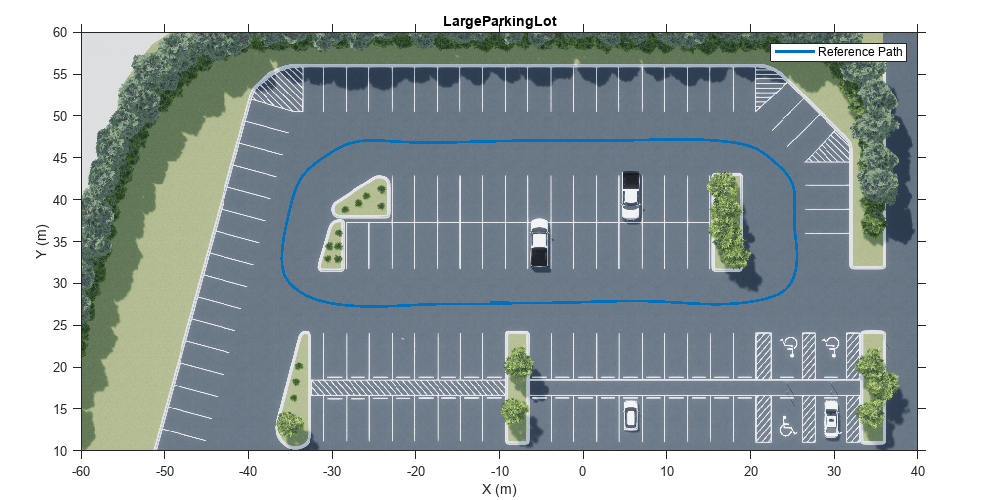After adding the ego vehicle, you can attach a camera sensor to it using the Simulation 3D Camera block. In this example, the camera is mounted on the left mirror of the ego vehicle with a rotation offset to point to the side of the vehicle. You can use the Camera Calibrator app to estimate intrinsics of the actual camera that you want to simulate.

```% Open the model modelName = "ParkingLaneMarkingsDetection"; open_system(modelName) % Set camera intrinsic parameters focalLength = [1109 1109]; % In pixels principalPoint = [401 401]; % In pixels imageSize = [801 801]; % In pixels```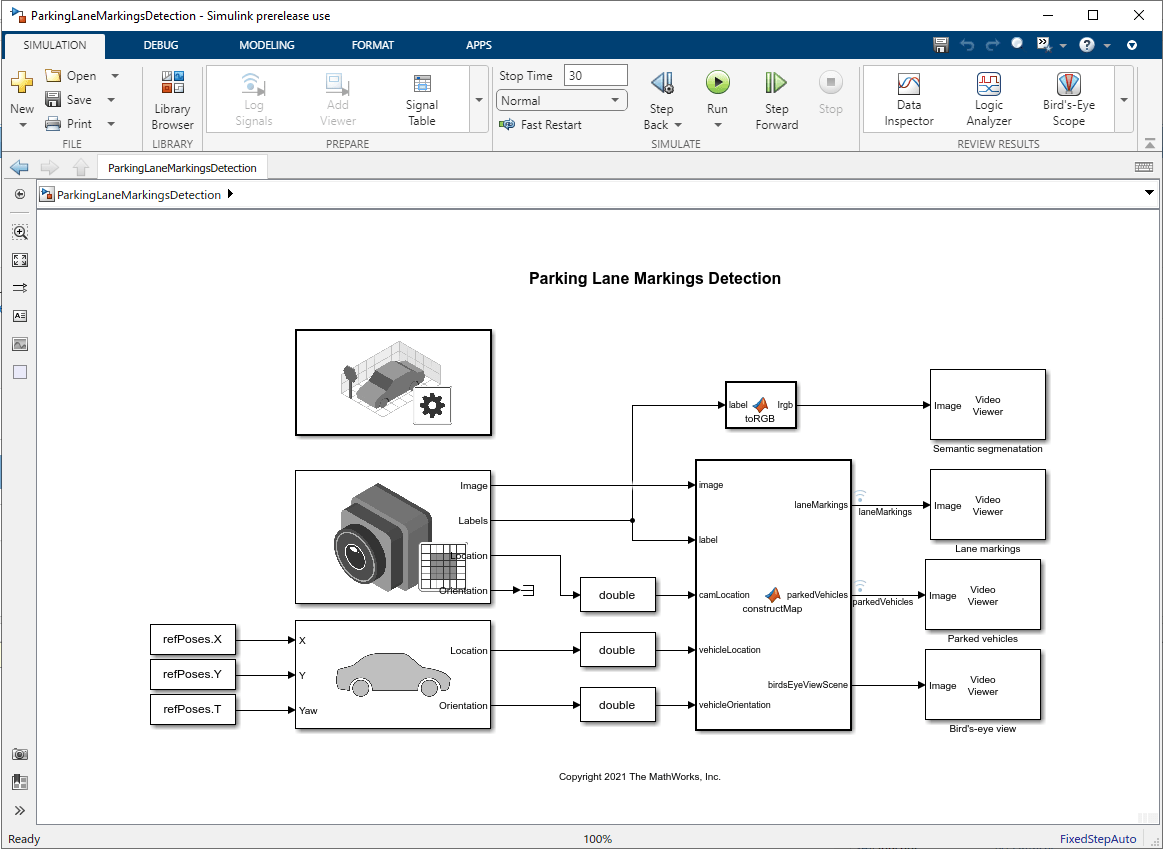### Build a Map

Using the camera mounted on the vehicle, the c`onstructMap` MATLAB Function block in the `ParkingLaneMarkingsDetection` model implements the algorithm to build a map, using these steps:

1. Detects parking lane markings and parked vehicles using semantic segmentation. For simplicity, this example uses the ground truth segmentation data from the `Label` outport of the Simulation 3D Camera block. In a more realistic implementation, you can replace this with a semantic segmentation algorithm to detect vehicles and lane markings from camera images.

2. Transforms detections from the image coordinates to the vehicle coordinates by applying a projective transformation using the `transformImage` object function of the `birdsEyeView` object.

3. Transforms detections from the local vehicle coordinates to the world coordinates using vehicle odometry. This example relies on the odometry provided by the ground truth of the Simulation 3D Vehicle with Ground Following block. In real applications, you can obtain this information from a localization subsystem that uses onboard IMU, wheel encoders, camera, lidar sensor, and any other sensors that help with accurate vehicle trajectory estimation. For an example of how to develop a visual localization system using synthetic image data in the Unreal Engine® simulation environment, see the Visual Localization in a Parking Lot example.

4. Builds a bird's-eye-view map of the parking lot by incrementally merging the detections in the world coordinates. The map consists of two layers represented by two binary images, `laneMarkings` and `parkedVehicles`. `parkedVehicles` contains the parked vehicles in the scene, representing obstacles. `laneMarkings` contains the parking lane markings used to determine the locations of parking spots.

```if ~ispc error(["3D Simulation is only supported on Microsoft", char(174), "Windows", char(174), "."]); end % Simulate the model sim(modelName);```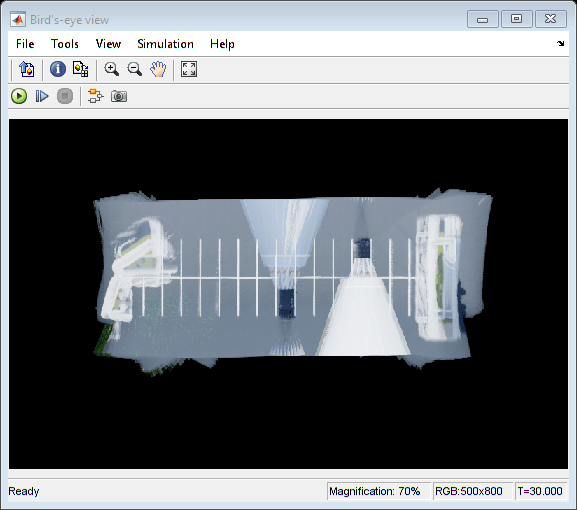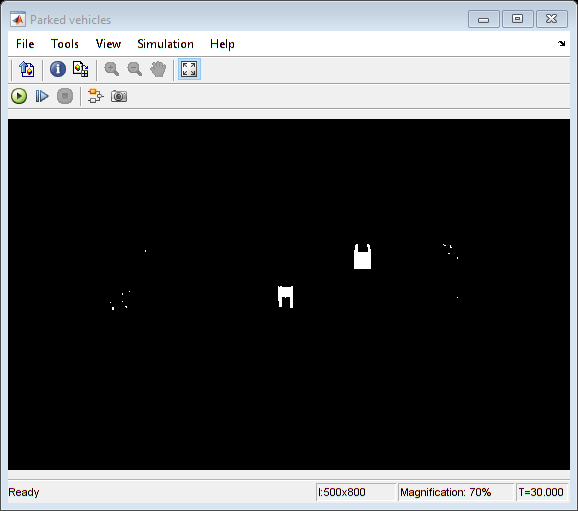### Detect Parking Lines

Parking spots are generally constructed using fixed-width, parallel, line segments. You can detect these line segments from the parking line markings by using the Hough transform. The `helperFindParkingLines` function extracts line segments based on the Hough transform and returns horizontal or vertical lines.

```% Get the data at the end of the simulation laneMarkings = logsout{1}.Values.Data(:,:,end); parkedVehicles = logsout{2}.Values.Data(:,:,end); % Close the model close_system(modelName) % Find parking lanes [parkingLines,isHorizontal] = helperFindParkingLines(laneMarkings); % Display parking lines helperPlotMap(parkingLines,laneMarkings,parkedVehicles);```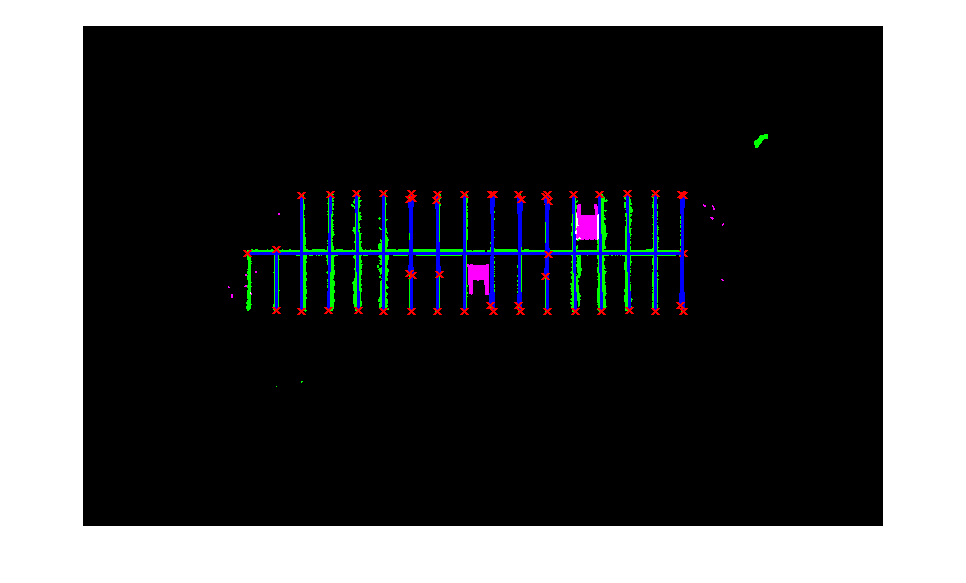The returned line segments may contain multiple lines that belong to the same line markings. To remove redundant lines, the `helperFindUnqiueLanes` function clusters the detected lines based on their orientations and center point positions, and keeps only the longest line in each cluster.

```% Collect features for each detected lane parkingLines = helperFindUnqiueLanes(parkingLines, isHorizontal); % Display the filtered lines ax = helperPlotMap(parkingLines,laneMarkings,parkedVehicles);```### Determine Empty Parking Spots

Next, find the empty parking spots by exploring all the vertices resulting from the detected lines and checking which ones could result in a rectangle with required dimensions. The vertices include both the starting and ending points of the detected lines, as well as the intersection points of the lines.

```% Find all the vertices vertices = helperGetVertices(parkingLines); % Display all the vertices plot(ax,vertices(:,1),vertices(:,2),"x",LineWidth=2,Color="red");```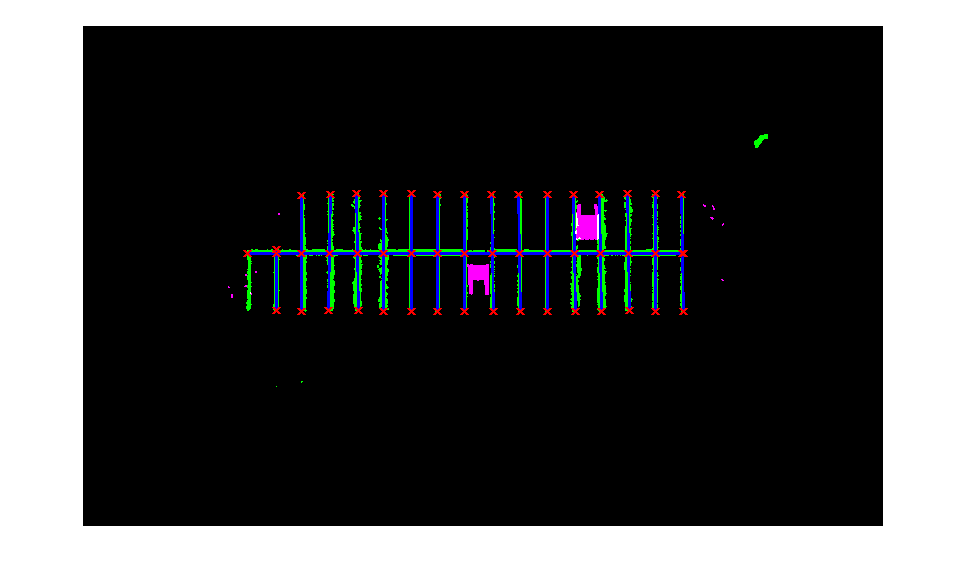To determine if a parking spot is empty, check if the convex hull of its four vertices overlaps with the obstacle area in `parkedVehicles`.

```% Identify parking spots based on area [parkingSpots,isOccupied] = helperFindParkingSpots(vertices,parkedVehicles); % Display empty parking spots on the map h1 = plot(ax, parkingSpots(~isOccupied),LineWidth=2,FaceColor="c",DisplayName="Empty spots"); % Display occupied parking spots on the map h2 = plot(ax, parkingSpots(isOccupied),LineWidth=2,FaceColor="r",DisplayName="Occupied spots"); % Add legends legend([h1(1) h2(2)])```After obtaining the locations of empty parking spots, you can execute a parking maneuver to park the vehicle. To learn how to plan a trajectory in a parking lot, see the Visualize Automated Parking Valet Using Unreal Engine Simulation example.

### Helper Functions

`helperFindParkingLines` finds lines from semantic segmentation results.

```function [lanes,isHorizontal] = helperFindParkingLines(map) [H,T,R] = hough(map); P = houghpeaks(H,150,Threshold=0.1*max(H(:))); lines = houghlines(map,T,R,P,FillGap=10,MinLength=50); lanes = [vertcat(lines.point1),vertcat(lines.point2)]; isHorizontal = abs(lanes(:, 1) - lanes(:, 3)) < 4; isVertical = abs(lanes(:, 2) - lanes(:, 4)) < 4; lanes = lanes(isHorizontal | isVertical,:); isHorizontal = isHorizontal(isHorizontal | isVertical); end```

`helperPlotMap` plots the occupancy map based on the data captured from the simulation.

```function ax = helperPlotMap(parkingLanes,binaryLanesMap,binaryCarsMap) occupancyMap = imfuse(binaryLanesMap,binaryCarsMap); figure ax = gca; imshow(occupancyMap,Parent=ax); hold(ax, 'on'); for i=1:size(parkingLanes,1) xy=parkingLanes(i,:); xy=[xy(1:2);xy(3:4)]; plot(ax,xy(:,1),xy(:,2),LineWidth=2,Color="blue"); plot(ax,xy(1,1),xy(1,2),"x",LineWidth=2,Color="red"); plot(ax,xy(2,1),xy(2,2),"x",LineWidth=2,Color="red"); end end```

`helperGetVertices` constructs a set of vertices using the end-points of the lines and their intersections.

```function vertices = helperGetVertices(lanes) vertices=[lanes(:,1:2); lanes(:,3:4)]; for i = 1:size(lanes,1) for j = i:size(lanes,1) point = helperFindLineIntersections(lanes(i,1:2),lanes(i,3:4),lanes(j,1:2),lanes(j,3:4)); if ~isempty(point) vertices = [vertices; point]; end end end vertices = unique(vertices,"rows"); end```

`helperFindUnqiueLanes` calculates a set of features for each lane.

```function uniqueLanes = helperFindUnqiueLanes(lanes,isHorizontal) % Cluster lines based on orientation and length startPoints = lanes(:,[1 2]); endPoints = lanes(:,[3 4]); numLanes = size(lanes,1); isLongest = true(numLanes,1); for i = 1:numLanes-1 for j = i+1:numLanes isPointsClose = norm(startPoints(i,:)-startPoints(j,:)) < 10 || norm(endPoints(i,:)-endPoints(j,:)) < 10 || ... norm(startPoints(i,:)-endPoints(j,:)) < 10 || norm(startPoints(i,:)-endPoints(j,:)) < 10; isSameOrientation = isHorizontal(i) & isHorizontal(j); if isPointsClose && isSameOrientation if norm(startPoints(i,:)-endPoints(i,:)) < norm(startPoints(j,:)-endPoints(j,:)) isLongest(i) = false; else isLongest(j) = false; end end end end uniqueLanes = lanes(isLongest,:); end```

`helperFindParkingSpots `finds parking spots constructed by parking lines

```function [parkingSpots,isOccupied] = helperFindParkingSpots(points,binaryCarsMap) % Check all the combinations of four points groups = nchoosek(1:size(points,1),4); numSpots = 1; spotArea = 1500; % Expected parking spot area for i = 1:size(groups,1) % Compute the distance between the center point and the four corner % points. If the distances are approximately the same, then the shape % constructed by the four corner points are a rectangle. cornerPoints = points(groups(i,:),:); centerPoint = mean(cornerPoints); distances = vecnorm(cornerPoints - centerPoint,2,2); hasCollinearPoints = numel(unique(cornerPoints(:,1)))==1 || ... numel(unique(cornerPoints(:,2)))==1; if max(distances) - min(distances) < 5 && ~hasCollinearPoints % Compute the area of the rectangle pgon = polyshape(cornerPoints,KeepCollinearPoints=true,Simplify=false); if numsides(pgon) == 4 hull = convhull(pgon); if abs(area(hull) - spotArea) < 500 mask = poly2mask(hull.Vertices(:,1),hull.Vertices(:,2), ... size(binaryCarsMap,1),size(binaryCarsMap, 2)); % Check if the spot is occupied by a parking vehicle parkingSpots(numSpots) = hull; %#ok<*AGROW> isOccupied(numSpots) = sum(sum(mask & binaryCarsMap))>100; numSpots = numSpots+1; end end end end end```

`helperFindLineIntersections` finds the intersection points of two lines.

```function point = helperFindLineIntersections(startPoint1,endPoint1,startPoint2,endPoint2) % Line1 : a1*x + b1*y = c1 a1 = endPoint1(2) - startPoint1(2); b1 = startPoint1(1) - endPoint1(1); c1 = a1 .* startPoint1(1) + b1 .* startPoint1(2); % Line2 : a2*x + b2*y = c2 a2 = endPoint2(2) - startPoint2(2); b2 = startPoint2(1) - endPoint2(1); c2 = a2 .* startPoint2(1) + b2 .* startPoint2(2); determinant = a1*b2 - a2*b1; point = []; if abs(determinant) > sqrt(eps(class(determinant))) % Two lines are not parallel point(1) = (b2*c1 - b1*c2)/determinant; point(2) = (a1*c2 - a2*c1)/determinant; % Check if the intersection point lies with the two line segments isValid = point(1) >= min(startPoint1(1), endPoint1(1)) && ... point(1) <= max(startPoint1(1),endPoint1(1)) && ... point(2) >= min(startPoint1(2),endPoint1(2)) && ... point(2) <= min(startPoint1(2),endPoint1(2)); if ~isValid point = []; end end end```